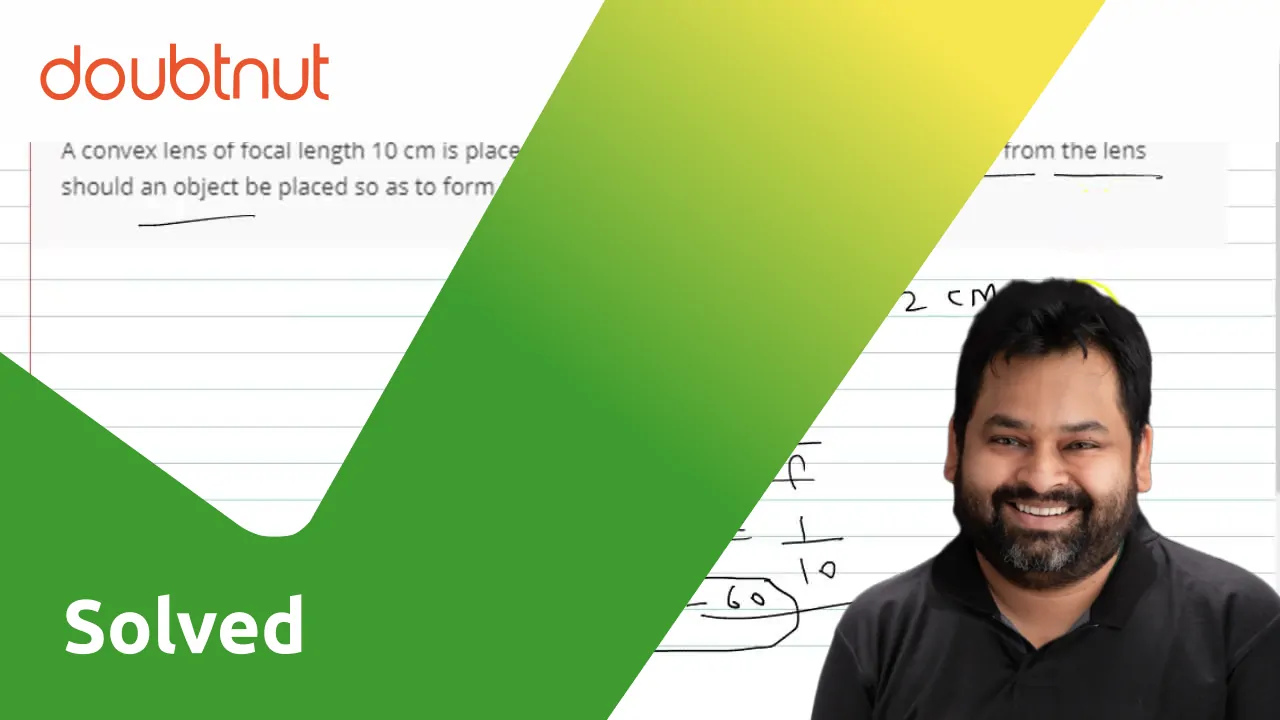Doubtnut App - Get 50 Lakh+ video solutions better than GoogleInstall Now

# A convex lens of focal length 10 cm is placed at a distance of 12 cm from a wall. How far from the lens should an object be placed so as to form its real image on the wall?

Text Solution
Verified by Experts

## Here, the real image is formed on the wall which is at a distance of 12 cm from the convex lens. This means that the distance of image from the convex lens or image distance will be 12 cm. Since a real image is fomred on the right side of the lens, so this image distance will be positive. Now, Image distance, v=±12 cm (A real image) Object distance, u=? (To be calculated) Focal length, f=+10 cm (it is convex lens) Putting these values in the lens formula: 1v−1u=1f We get: 112−1u=110 112−110=1u 5−660=1u −160=1u So, Object distance, u=-60 cm Thus, the obect should be place at a distance of 60 cm in front of the convex lens. The minus sign shows that the obect is on the left side of the lens.